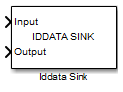Documentation

Iddata Sink

Export simulation data as iddata object to MATLAB workspace

• Library:
• System Identification ToolboxDescription

The Iddata Sink block exports simulation data as an iddata object to the MATLAB® workspace. The object stores the input and simulated output signals, sampled at the sample time that you specify. If you simulate your model from the model window, the block exports the object to the MATLAB base workspace. If you simulate the model programmatically, the object is exported to the MATLAB caller workspace. The caller workspace is the workspace of the function that called the currently running function.

Ports

Input

expand all

Input of iddata object, specified as a scalar for single-input data. For multichannel data with Nu inputs, specify Input as a vector of length Nu.

Data Types: double

Output of iddata object, specified as a scalar for single-output data. For multichannel data with Ny outputs, specify Output as a vector of length Ny.

Data Types: double

Parameters

expand all

Name of iddata object, specified as a MATLAB variable name. The object is exported with this name to the MATLAB workspace.

Sample time in seconds, specified as a finite positive number. The iddata object stores the input and output signals, sampled at the sample time that you specify.